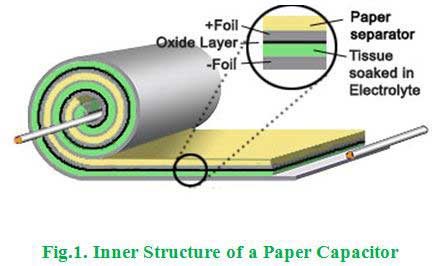# MCQs on Electric Circuits

##### Page 32 of 63. Go to page 1 2 3 4 5 6 7 8 9 10 11 12 13 14 15 16 17 18 19 20 21 22 23 24 25 26 27 28 29 30 31 32 33 34 35 36 37 38 39 40 41 42 43 44 45 46 47 48 49 50 51 52 53 54 55 56 57 58 59 60 61 62 63
01․ A capacitor consists of
two insulator separated by a conductor.
two conductors seperated by an insulator.
two insulator only.
two conductor only.

The basic construction of the capacitor is that consists of two parallel metal plates separated by an insulating material or the dielectric material. For example, air capacitors are made with air as the dielectric in between the two metal plates or conductors.

02․ Which capacitor will be physically smaller for the same ratings?
Ceramic capacitor.
Paper capacitor.
Both will be equal size.
None of them.

The dielectric strength of ceramic material is very high than the paper dielectric material. Hence, the dielectric strength and the dimension are inversely proportional. That means the higher dielectric material occupies less space to produce the required rating than the other materials. So, the physical size of the ceramic capacitor is less for the same rating than the paper capacitor.

03․ A paper capacitor is usually available in form of
tubes.
rolled foil.
disc.
meshed plates.

Paper capacitors are made of paper or oil-impregnated paper and aluminum foil layers rolled into a cylinder as shown in below figure.04․ A capacitor in a circuit became hot and ultimately exploded due to wrong connection, which type of capacitor it could be?
Paper capacitor.
Ceramic capacitor.
Electrolytic capacitor.
Any of above.

The electrolytic capacitors are polarized. So, when the DC voltage applied to the capacitor, the terminals must connect with the correct polarity, i.e. positive power supply to the positive terminal and negative power supply to the negative terminal. If there is any incorrect polarization, the insulating oxide layer will break down very soon and it causes permanent damage as a result.

05․ A ceramic and a mica capacitor have the same physical dimension. Which has the higher value of capacitance?
Mica capacitor.
Ceramic capacitor.
Both have identical value of capacitor.
It is impossible to conclude on the basis of information supplied.

Mica material has a dielectric strength of 5000 KV per inch. This is greater than the ceramic material. At the same physical dimensions of both two materials due to the difference in dielectric strength, the mica capacitor has the higher value of capacitance than the ceramic capacitor because the dielectric strength and the capacitance are directly proportional to each other.

06․ A condenser suitable for DC only is
metallic plate variable gang condenser.
poled aluminium electrolytic condenser.
oil impregrated paper condenser.
none of above.

The polymer aluminium electrolytic condenser is a polarized capacitor that can be worked only in DC supply and the charging and discharging characteristics are very good than the other above mentioned capacitors. Hence, it is suitable for DC power supply.

07․ Which of following capacitors preferred for high frequency circuits?
Air capacitor.
Electrolytic capacitor.
Glass capacitor.
Mica capacitor.

Mica capacitors have low resistive and inductive components associated with it. Hence, they have high Q factor and because of high Q factor their characteristics are mostly frequency independent, which allows this capacitor to work at high frequency.

08․ ________________ capacitors are used in transistor circuits.
Paper.
Ceramic.
Air.
Electrolytic.

The ceramic capacitor has the special characteristics that the capacitor only transmits AC components and does not transmit DC components, and it is used to extract AC components from DC + AC components. As the operating conditions of transistors, ICs and other active elements in the circuits, it is necessary to extract only the required AC signal after setting the optimal operating conditions for each circuit. That is why the ceramic capacitors are used in transistor circuits.

09․ Which of the following capacitors can be used for temperature compensation?
Ceramic capacitor.
Air capacitor.
Paper capacitor.
All of the above.

The low permittivity of ceramic capacitors can be made to exhibit zero temperature coefficient and this characteristics helps in temperature compensation in networks.

10․ Two wires of A and B of the same material and length ‘l’ and ‘2l’ have radius ‘r’ and ‘2r’ respectively. The ratio of their specific resistance will be
1:1.
1:2.
4:1.
1:8.

The specific resistance is independent of length and radius of wire and depends only on the material.

<<<3031323334>>>